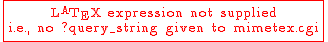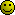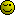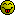Inscription / Connexion Nouveau Sujet
Niveau BTS
Partager :

# Pourcent d un produit

Posté par Percent (invité) 01-09-04 à 07:12Problem is that
T = P x C
Now make P increase by P% and C increase by C%
I need to know a formula of what would be
T% by using P% and C%.
( It is not C%+P%)

When you derive a % of variation you want to know how many percent of increase / decrease.
You have P1 the old price and P2 the new price and want to know how many % P1 was changed to have P2.
This is displayed as +/-CURR.PR%  Let's call that percentage P%

You do : P% = (P2 - P1) / P1
right ? Why ? You take your P1 and add the variation %

P2 = P1 + ( P1 x P% )/100
So P%  = (P2 - P1) / P1  ( remove the 100 of % )

Example :
You had 20 in your pocket and later 30. How many % increase ? You know it's 50%
50% of 20 is 10.
20 + 10 = 30

( 30-20)/20 = 1/2 = 0.5 makes 50%

Same for the currency exchange C%
C% = +/-EXCH.% =  (C2 - C1)/C1

In our case
AV.COST P.=100,01100 This is P1
CUR. PRICE = 98,4500 This is P2
So the % of change for PRICE is  (98,4500 - 100,011)  / 100,011 = -1.56

Same, the % of change for FX is
Current rate is (118,749.60 / 98,450 ) = C2 = 1.2062
AV. EXCH. = 1,1644 = C1

%C = (1.2062 - 1,1644 ) / 1,1644 = 3,58

The same way the 1.97 is computed. This is the global % of unrealised in valuation currency.
So you have an

- average purchase amount in USD that is ( AV.COST P x AV. EXCH. ) = P1 x C1 = 116.4528 Let's call it T1=P1 x C1
- At valuation you have CUR. PRICE x Current rate = P2 x C2 = 118.75039 Lett's call it T2= P2 x C2

So the % of increase from T1 to T2 is ...
+/-GROSS% = (T2 - T1) / T1
+/-GROSS% = [ (P2xC2) - ( P1xC1) ]  / ( P1xC1) = 1.97

And ... the global one is NOT ( %C + %P )
This is mathematics for a % of a result of a product.
You have T = P x C ( Price x Currency exchange ) where P moves of P% and C moves of C% the global move of T
is NOT P% + C%

re : Pourcent d un produit 01-09-04 à 10:35

T = P x C
Now make P increase by P% and C increase by C%
I need to know a formula of what would be
T% by using P% and C%.

I change the problem to avoid confusion.
The problem becomes:

T = P x C
Now make P increase by X % and C increase by  Y %
I need to know a formula of what would be:
Z% by using X % and  Y %. (With Z% the augmentation of T)
----

T*(1 + (Z/100)) =(P * (1 + (X/100)) * (C * (1 + Y/100))
P*C*(1 + (Z/100)) =(P * (1 + (X/100)) * (C * (1 + Y/100))
(1 + (Z/100)) = (1 + (X/100)) * (1 + Y/100))$Z/100 = (1 + (X/100)) * (1 + Y/100)) - 1$$Z = \frac{(100+X)(100+Y)}{100} - 100$$Z = \frac{10000+100X+100Y+XY}{100} - 100$$Z = \frac{100X+100Y+XY}{100}$
----

Numerical check

P = 125
C = 26

P increase of 5% -> X = 5
C increase of 7% -> Y = 7$Z = \frac{100X+100Y+XY}{100} = \frac{500+700+35}{100} = 12.35$
So T increase by 12.35 %

T = P*C = 125*26 = 3250
T' = (P*1,05) * (C*1,07) = 3651,375
which corresponds to (3621,375-3250)/3250 = 0,1235 = 12.35% -> OK.Posté par (invité)re : Pourcent d un produit 01-09-04 à 11:20

Thanks and I came to the same result.

We valuate a security ( stocks ... )
The security currency is EUR but we valuate in USD ( for example )
P1 price at date 1
C1 exchange EUR / USD rate at date 1
P2 price at date 2
C2 exchange rate at date 2

Value of security at date 1 T1=P1xC1
Value of security at date 2 T2=P2xC2

Now we see by what % the P1 is increased to get P2.
P2 is the price of P1 increased by P% %.
C2 is the exchange rate of C1 increased by C% %.

So the security valuations can be increased/decreased
by those 2 components,
- price itself in security currency
- exchange rate of the currency and the reporting
currency

P2 = P1 + (P1xP%)/100
P%= 100 x ( P2 - P1)/ P1 =  100 x ( P2/P1 - P1/P1 ) = 100 x [ ( P2/P1) - 1 ]
P% = 100 x [ ( P2/P1) - 1 ]
P2/P1 = (P%/100) + 1

Same C2/C1 = (C%/100) + 1

T% = 100 x [ ( T2/T1) - 1 ]
T% = 100x [ [ ( P2 C2 / P1 C1 ) ] - 1 ]
T% =100x [ P2/P1 C2/C1 ) -1 ]

We saw that P2/P1 = (P%/100) + 1
We saw that C2/C1 = (C%/100) + 1

T% =100 ([(P%/100) + 1] [(C%/100) + 1 - 1 )
T%= 100 ( P%C%/10000  + P%/100 + C%/100 + 1  -1 ]
So the way to get the consolidated % given the 2 split % is
T% = (P% C%)/100 + P% + C%

1.97 = (-1.56 x 3.58) - 1.56 + 3.58

Posté par (invité)re : Pourcent d un produit 01-09-04 à 11:47

Et ... desole de l'avoir mis en anglais mais
j'avais vu des posts ou les gens demandaient en
anglais bien que le site soit en francais.
Bonne journee.

re : Pourcent d un produit 01-09-04 à 13:42

Tiens si j'avais su, je t'aurais répondu dans mon patoi régional.$6 Ouf\ ti,\ ni\ fo\ ti\ nin\ aredji$

Posté par Nath63 (invité)re : Pourcent d un produit 01-09-04 à 15:50

Bonjour !

C'est qd même rare que des post soient écris en anglais, à ma mémoire , qui est bonne en général, Lol, j'en connais qu'un à ce jour !!

Sympa ton patoi de chez toi JpMoi c'est du patois d'auvergne hihiA+
Nathaliere : Pourcent d un produit 01-09-04 à 16:27

Salut Nath63

Moi c'est du Wallon de Liège. (Belgique pour ceux qui l'ignore)

Vous devez être membre accéder à ce service...

Pas encore inscrit ?

1 compte par personne, multi-compte interdit !

Ou identifiez-vous :

Inscription gratuite

Fiches en rapport

parmi 1674 fiches de maths

Désolé, votre version d'Internet Explorer est plus que périmée ! Merci de le mettre à jour ou de télécharger Firefox ou Google Chrome pour utiliser le site. Votre ordinateur vous remerciera !